In :
%pylab inline

Welcome to pylab, a matplotlib-based Python environment [backend: module://IPython.zmq.pylab.backend_inline].

In :
from pandas import *
import numpy as np
from sklearn.grid_search import GridSearchCV
import sklearn.cross_validation as cv
import sklearn.metrics as metrics
from sklearn.svm import l1_min_c
from sklearn.linear_model import Lasso, LassoCV, LogisticRegression
import scipy.linalg as la
from math import pi
import matplotlib.pyplot as plt
import statsmodels.api as sm
from statsmodels.formula.api import ols
from patsy import dmatrix
import re
import os
import math
from tdm_df import tdm_df


# Fitting sine wave data by polynomials¶

In :
sin_data = DataFrame({'x' : np.linspace(0, 1, 101)})
sin_data['y'] = np.sin(2 * pi * sin_data['x']) + np.random.normal(0, 0.1, 101)

In :
sin_linpred = ols('y ~ x', df = sin_data).fit().predict()

In :
plt.plot(sin_data['x'], sin_data['y'], 'k.')
plt.plot(sin_data['x'], sin_linpred, 'r-', label = 'Linear Model Prediction')
plt.title('Sine wave with Gaussian noise')
plt.legend(loc = 'upper left')

Out:
<matplotlib.legend.Legend at 0x10a728690>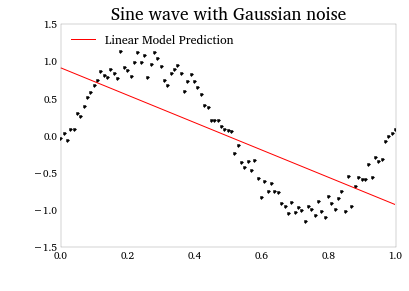In a polynomial regression, we want to approximate the data with by the sum of a finite number of orthonormal polynomial (OP) basis functions. In a regression setting, this means making the multiple regressors by computing the OP transformations of $x$. Then, we have:

$y(x_i) = \sum_{d=1}^{D}\mathcal{P}_d(x_i) + \varepsilon_i$

where $\mathcal{P}_d$ is the $d$th order OP basis functions, and $\varepsilon_i$ is random noise.

Fortunately, patsy has a method for computing OP transformations, via the dmatrix function.

In :
x = sin_data['x']
y = sin_data['y']
Xpoly = dmatrix('C(x, Poly)')
Xpoly1 = Xpoly[:, :2]
Xpoly3 = Xpoly[:, :4]
Xpoly5 = Xpoly[:, :6]
Xpoly25 = Xpoly[:, :26]

In :
polypred1 = sm.OLS(y, Xpoly1).fit().predict()
polypred3 = sm.OLS(y, Xpoly3).fit().predict()
polypred5 = sm.OLS(y, Xpoly5).fit().predict()
polypred25 = sm.OLS(y, Xpoly25).fit().predict()


Plotting the fits of polynomial models of increasing order, we see that by D = 3, we've pretty much captured the salient features of the data, and much beyond that we're probably over-fitting.

In :
plt.figure(figsize(10, 8))
fig, ax = plt.subplots(2, 2, sharex = True, sharey = True)
fig.subplots_adjust(hspace = 0.0, wspace = 0.0)
fig.suptitle('Polynomial fits to noisy sine data', fontsize = 16.0)

# Iterate through panels (a), model predictions (p), and the polynomial
# degree of the model (d). Plot the data, the predictions, and label
# the graph to indicate the degree used.
for a, p, d in zip(ax.ravel(), [polypred1, polypred3, polypred5, polypred25],
['1', '3', '5', '25']):
a.plot(x, y, '.', color = 'steelblue', alpha = 0.5)
a.plot(x, p)
a.text(.5, .95, 'D = ' + d, fontsize = 12,
verticalalignment = 'top',
horizontalalignment = 'center',
transform = a.transAxes)
a.grid()

# Alternate axes that have tick labels to avoid overlap.
plt.setp(fig.axes.get_yaxis().get_ticklabels(), visible = False)
plt.setp(fig.axes.get_yaxis(), ticks_position = 'right')
plt.setp(fig.axes.get_xaxis(), ticks_position = 'top')
plt.setp(fig.axes.get_xaxis().get_ticklabels(), visible = False)

Out:
[None, None, None, None, None, None]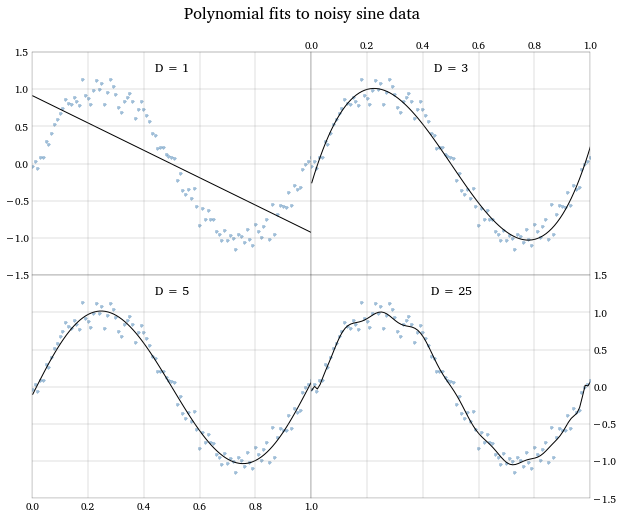Just for shits and giggles, a home-rolled function to build the orthonormal-polynomial basis functions from a vector. This will test we understand what Poly does. If you check it, you'll find this function and Poly provide the same results.

In :
def poly(x, degree):
'''
Generate orthonormal polynomial basis functions from a vector.
'''
xbar = np.mean(x)
X = np.power.outer(x - x.mean(), arange(0, degree + 1))
Q, R = la.qr(X)
diagind = np.subtract.outer(arange(R.shape), arange(R.shape)) == 0
z = R * diagind
Qz = np.dot(Q, z)
norm2 = (Qz**2).sum(axis = 0)
Z = Qz / np.sqrt(norm2)
Z = Z[:, 1:]
return Z

In :
np.random.seed(1)
rand_ind = arange(100)
np.random.shuffle(rand_ind)
train_ind = rand_ind[:50]
test_ind = rand_ind[50:]


## A polynomial fit to the sine wave data using lasso regularization¶

In the book, the authors set up an explicit cross-validation scheme, by separating the data into training and testing sets. For a series of candidate lasso penalty parameters, they fit the model on the training data, then evaluate the MSE of that model on the testing data.

We're going to use sklearn's LassoCV, which automatically performs cross-validation. The function return the optimal lasso penalty parameter across the CV folds (i.e., the one that minimizes the average MSE across the folds).

Some notes: sklearn calls its lasso penalty parameter alpha, compared to R's glmnet which calls it lambda. (I'm more accustomed to the latter, myself). This can get confusing, because glmnet also has an alpha parameter that weights the L1 and L2 cost functions.

Also, sklearn seems to weight its L1 cost function differently than glmnet leading to different values for the lasso parameter. I haven't looked closely yet at the source of the discrepancy. The other model parameters and the model predictions, though, should be roughly the same for both sklearn's Lasso and glmnet.

In :
lasso_model = LassoCV(cv = 15, copy_X = True, normalize = True)
lasso_fit = lasso_model.fit(Xpoly[:, 1:11], y)
lasso_path = lasso_model.score(Xpoly[:, 1:11], y)

In :
# Plot the average MSE across folds
plt.plot(-np.log(lasso_fit.alphas_), np.sqrt(lasso_fit.mse_path_).mean(axis = 1))
plt.ylabel('RMSE (avg. across folds)')
plt.xlabel(r'$-\log(\lambda)$')
# Indicate the lasso parameter that minimizes the average MSE across folds.
plt.axvline(-np.log(lasso_fit.alpha_), color = 'red')

Out:
<matplotlib.lines.Line2D at 0x10a43fed0>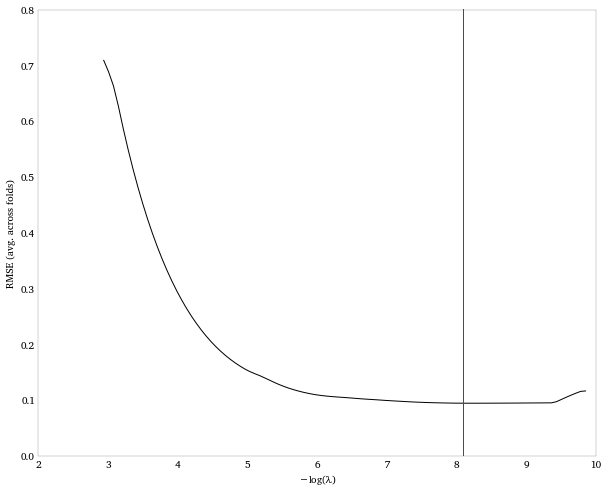If we look at the resulting coefficients, we see that the lasso shrunk nearly all the coefficients to zero except those on the first and third polynomial. This makes sense, since we ought to be able to capture all the important features of a sine wave on [0, 1] using just a third degree polynomial, as we saw in the chart above.

In :
print 'Deg.  Coefficient'
print Series(np.r_[lasso_fit.intercept_, lasso_fit.coef_])

Deg.  Coefficient
0    -0.003584
1    -5.359452
2     0.000000
3     4.689958
4    -0.000000
5    -0.547131
6    -0.047675
7     0.124998
8     0.133224
9    -0.171974
10    0.090685


As we'd expect this simplified model does a good job of fitting the data without over-fitting.

In :
plt.plot(x, y, '.')
plt.plot(np.sort(x), lasso_fit.predict(Xpoly[:, 1:11])[np.argsort(x)],
'-r', label = 'Training data lasso fit')

Out:
[<matplotlib.lines.Line2D at 0x10a463290>]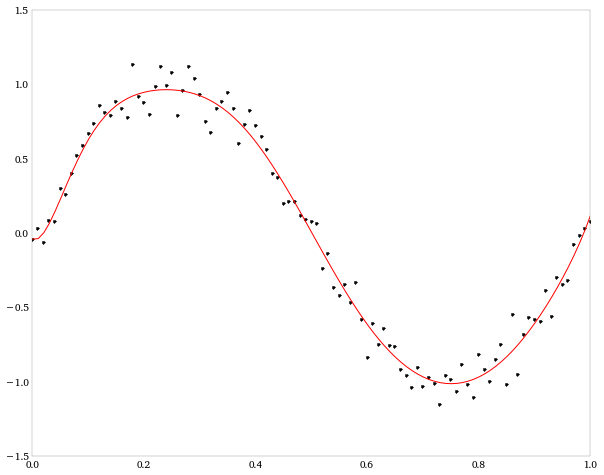# Predicting O'Reilly book sales using back-cover descriptions¶

In :
ranks_df = read_csv('data/oreilly.csv')
ranks_df.columns = ['ipfamily', 'title', 'isbn', 'rank', 'long_desc']

           ipfamily                                 title           isbn  rank  \
0  9780596000271.IP                  Programming Perl, 3E  9780596000271     1
1  9781565923928.IP  Javascript: The Definitive Guide, 3E  9781565923928     2
2  9780596007126.IP            Head First Design Patterns  9780596007126     3
3  9780596009205.IP                   Head First Java, 2E  9780596009205     4
4  9780596529529.IP  Mac OS X Leopard: The Missing Manual  9780596529529     5

long_desc
0  Perl is a powerful programming language that  ...
1  JavaScript is a powerful scripting language th...
2  You're not alone.<br />\r<br />\rAt any given ...
3  Learning a complex new language is no easy tas...
4  With Leopard, Apple has unleashed the greatest...

In :
# Reverse the ranks, so that 100 is the best seller, and 1 is the worst.
ranks = ranks_df['rank']
rank_rev_map = {i: j for i, j in zip(ranks, ranks[::-1])}
ranks = ranks.replace(rank_rev_map)

docs = ranks_df['long_desc']


## Creating a term-document matrix from the descriptions¶

Looking at a sample document (back-cover description), we see that there is some html markup we should strip out before processing.

In :
print docs

This third edition of <i>Programming Perl</i> has been expanded to cover version 5.6 of this maturing language. New topics include threading, the compiler, Unicode, and other  new features that have been added since the previous edition.

In :
tag_pattern = r'<.*?>'
docs_clean = docs.str.replace(tag_pattern, '')

In :
print docs_clean

This third edition of Programming Perl has been expanded to cover version 5.6 of this maturing language. New topics include threading, the compiler, Unicode, and other  new features that have been added since the previous edition.


To create the term-document matrix, we'll use the tdm_df function we created in chapter 3, and used again in chapter 4. Again, for comparability with the book, we'll use the stopwords from R's tm package.

In :
rsw = read_csv('../ch3/r_stopwords.csv', squeeze = True).tolist()
desc_tdm = tdm_df(docs_clean, stopwords = rsw)


## Lasso regression¶

Our goal is to predict the rank of book $i$, based on what terms appear in its description. That is,

$y_i = \beta_0 + \beta_1 x_{1i} + \beta_2 x_{2i} + \ldots + \beta_K x_{Ki} + \varepsilon_i$

where $x_{ki}$ is the number of times that term $k$ appears in the description of book $i$.

Let's fit the model with the lasso regularizer, using LassoCV to find the best value of the lasso parameter. Again, we'll let LassoCV do our cross-validation, instead of doing the explicit training-testing evaluation in the book.

Note: We're running 10 CV folds, which can be a bit slow to run. If it's too slow, it's ok to turn down the value. This also may throw a convergence warning sometimes.

In :
lasso_model = LassoCV(cv = 10)
lasso_fit = lasso_model.fit(desc_tdm.values, ranks.values)


If we look at the RMSE from this model, we can see we don't do a very good job. The regularizer essentially doesn't find any of the covariates worth keeping.

In :
plt.plot(-np.log(lasso_fit.alphas_), np.sqrt(lasso_fit.mse_path_), alpha = .5)
plt.plot(-np.log(lasso_fit.alphas_), np.sqrt(lasso_fit.mse_path_).mean(axis = 1),
lw = 2, color = 'black')
plt.ylim(0, 60)
plt.xlim(0, np.max(-np.log(lasso_fit.alphas_)))
plt.title('Lasso regression RMSE')
plt.xlabel(r'$-\log(\lambda)$')
plt.ylabel('RMSE (and avg. across folds)')

Out:
<matplotlib.text.Text at 0x110794e90>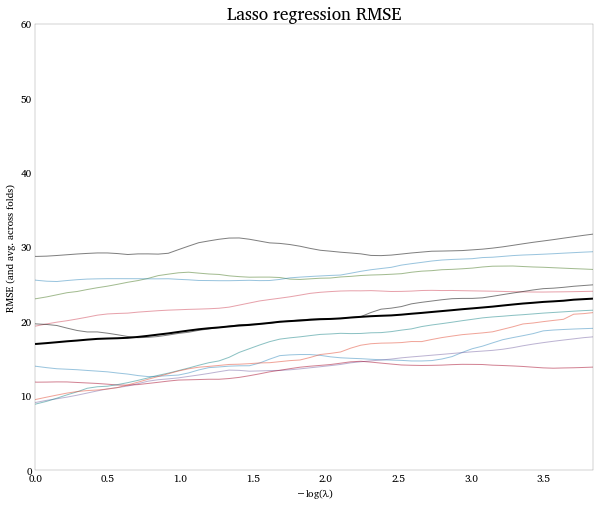## Logistic regression¶

Let's simplify the rank data, so instead of trying to predict the rank, we simply try to predict whether or not a book is in the top-50 sellers. This binary classification let's use a logistic regression.

Scikits-learn let's us use an L1-regularizer (lasso) with our logistic model. But it doesn't provide an automatic CV method. So we'll set up an explicit cross-validation, similar to what's done in the book.

The lasso penalty parameter in the LogisticRegression function is called C. The smaller C is, the larger the penalty.

For a given set of data, there is a minimum C, below which, the regularizer will set all the model parameters to zero. There's no point in use evaluating the fit of models for values of C less than this minimum. The function l1_min_c computes this minimum.

In :
# Create the TDM.
desc_tdm = tdm_df(docs_clean, stopwords = rsw)

# Make the rank variable binary, indicating Top 50 status.
top50 = 1 * (ranks_df['rank'] <= 50)

# Compute the minimum value of the L1 penalty parameter. Below this
# value, all model parameters will be shrunk to zero.
min_l1_C = l1_min_c(desc_tdm.values, top50.values)

# Create a space of penalty parameters to test.
cs = min_l1_C * np.logspace(0, 3, 10)

# Create a dictionary whose keys are the candidate values of C.
# The dictionary will hold the error rates in each CV trial for that
# value of C.
cdict = {}
for c in cs:
cdict[c] = []

# Add the target variable to the data, so it gets shuffled and split along
# with the predictor data.
desc_tdm['Top50'] = top50

# Set up logistic model
logit_model = LogisticRegression(C = 1.0, penalty = 'l1', tol = 1e-6)


We want to split our data into training and testing sets. We created a function to do this in chapter 4. Let's just define it again here.

In :
def train_test_split(df, train_fraction = .5, shuffle = True,
preserve_index = True, seed = None):
'''
Split a dataframe into training and test sets by randomly assigning
its observations to one set or the other.

Parameters
----------
df: a DataFrame
train_fraction: the fraction of df to be assigned to the
training set (1-train_fraction will go to the test set).
preserve_index: If True, the split dataframes keep their index
values from df. If False, each set gets a new integer index
of arange(# rows).
seed: the random number generator seed for row shuffling.
'''
if seed:
random.seed(seed)

nrows = df.shape
split_point = int(train_fraction * nrows)

rows = range(nrows)
if shuffle:
random.shuffle(rows)

train_rows = rows[:split_point]
test_rows  = rows[split_point:]

train_index = df.index[train_rows]
test_index  = df.index[test_rows]

train_df = df.ix[train_index, :]
test_df  = df.ix[test_index, :]

if not preserve_index:
train_df.index = np.arange(train_df.shape)
test_df.index  = np.arange(test_df.shape)

return train_df, test_df


Here's the cross-validation procedure. For 50 folds, we'll randomly split the data into training and testing sets. Then for each candidate value of the penalty parameter C, we'll fit the model on the training data, and compute its error rate in the testing data. The error rate is the proportion of books in the test data that the model misclassifies.

At the end we'll have 50 error rate curves (where the curve is over values of C). Then we'll compute the average error rate, for each candidate C, across the 50 trials.

In :
np.random.seed(4)
for i in xrange(50):
# Split the data
train_docs, test_docs = train_test_split(desc_tdm, .8)
trainy, testy = train_docs.pop('Top50'), test_docs.pop('Top50')
trainX, testX = train_docs, test_docs

# Fit the model for each candidate penalty parameter, C.
# then compute the error rate in the test data and add
# it to the dictionary entry for that candidate.
for c in cdict:
logit_model.set_params(C=c)
logit_fit = logit_model.fit(trainX.values, trainy.values)
predy = logit_fit.predict(testX.values)
error_rate = np.mean(predy != testy)
cdict[c].append(error_rate)


Plotting mean error rates across penalty parameter candidates, we find an optimal value of C around 0.15.

In :
error_path = DataFrame(cdict).mean()
error_path.plot(style = 'o-k', label = 'Error rate')
error_path.cummin().plot(style = 'r-', label = 'Lower envelope')
plt.xlabel('C (regularization parameter)')
plt.ylabel('Prediction error rate')
plt.legend(loc = 'upper right')

Out:
<matplotlib.legend.Legend at 0x1135df350>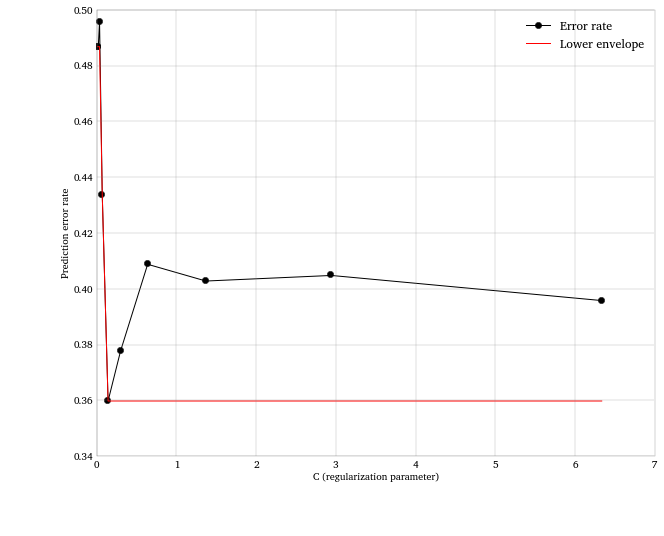We'll re-fit the model at the optimal C, and check out what terms the lasso keeps in the model. If turns out we keep about 9 or so terms. (This will change if you change the seed above, since it depends on the randomized CV folds).

These are the terms that are most informative about whether a book is a Top 50 seller. What are they?

In :
# If you run this block, you have to re-run the blocks starting from four above
# this block (it starts with the comment '# Create the TDM'). Otherwise the
# attempt to pop Top50 will throw an error ('cause you can't pop it twice).

min_error_c = error_path.index[error_path.argmin()]
logit_model_best = LogisticRegression(C = min_error_c, penalty = 'l1')

ally = desc_tdm.pop('Top50')
allX = desc_tdm

logit_fit_best = logit_model_best.fit(allX.values, ally.values)
keep_terms = desc_tdm.columns[np.where(logit_fit_best.coef_ > 0)]

print Series(keep_terms)

0          unix
1          perl
2          html
3        server
4          java
5         plsql
6          palm
7            os
8           awk
9    javascript


## Using scikit-learn's built-in cross-validation functions¶

scikit-learn has a built-in function for finding model hyper-parameters by cross-validation, as we did above, called GridSearchCV.

Here we'll perform the CV differently than the authors do in the book and rely more on built-in scikit-learn functions to do so.

First, we'll split the data into an 80-observation training set and a 20-observation test set using the train_test_split function from the sklearn.cross_validation module (instead of the handmade one we used above).

Second, we'll use GridSearchCV to perform CV fits for each value of the regularization parameter we supply. To choose the parameter to minimize the error rate, we'll maximize the zero_one_score function from the sklearn.metrics module. The result of this function is equal to 1 minus the error rate we were using above.

Finally, we'll fit this CV-optimized model on the 20 test observations, and use scikit-learn's other metrics functions to assess the model fit on the test set.

First, split the data into test and training sets. Then define the array of parameter values we'll grid-search over, and the number of CV folds we want to use in the search process.

In :
trainX, testX, trainy, testy = cv.train_test_split(
allX, ally, test_size = 0.2)

# Dictionary holding parameters to perform a grid-search over,
# and the arrays of values to use in the search.
c_grid = [{'C': cs}]

# No. of CV folds
n_cv_folds = 20


Define and fit the model.

In :
clf = GridSearchCV(LogisticRegression(C = 1.0, penalty = 'l1'), c_grid,
score_func = metrics.zero_one_score, cv = n_cv_folds)
clf.fit(trainX, trainy)

Out:
GridSearchCV(cv=20,
estimator=LogisticRegression(C=1.0, class_weight=None, dual=False, fit_intercept=True,
intercept_scaling=1, penalty='l1', tol=0.0001),
fit_params={}, iid=True, loss_func=None, n_jobs=1,
param_grid=[{'C': array([ 0.00633,  0.01364,  0.02938,  0.06329,  0.13636,  0.29377,
0.63291,  1.36357,  2.93771,  6.32911])}],
pre_dispatch='2*n_jobs', refit=True,
score_func=<function zero_one_score at 0x108eaf668>, verbose=0)

What is the optimal regularization parameter chosen by the grid search? And what is the error-rate for this optimized model on the training data?

We get similar results to the authors, even though our CV procedure is different

In :
clf.best_params_, 1.0 - clf.best_score_

Out:
({'C': 0.29377144516536563}, 0.375)

As we did above, let's plot the training data error rates vs. candidates for the regularization parameter. Since fitting the GridSearchCV function provided the output for each CV fold, we can add standard errors around the average error rate.

In :
rates = np.array([1.0 - x for x in clf.grid_scores_])
stds   = [np.std(1.0 - x) / math.sqrt(n_cv_folds) for x in clf.grid_scores_]

plt.fill_between(cs, rates - stds, rates + stds, color = 'steelblue', alpha = .4)
plt.plot(cs, rates, 'o-k', label = 'Avg. error rate across folds')
plt.xlabel('C (regularization parameter)')
plt.ylabel('Avg. error rate (and +/- 1 s.e.)')
plt.legend(loc = 'best')
plt.gca().grid()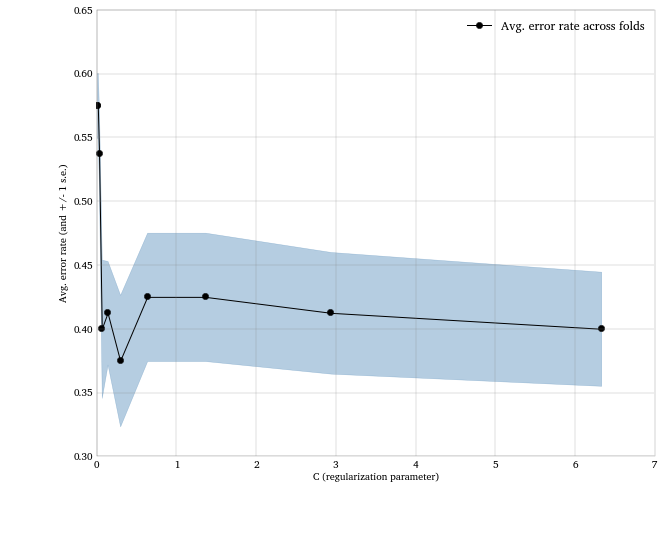We can use the predict method to fit this optimized model on the test-set inputs. Then, we can use the classification_report and confusion_matrix functions to assess the fit on the test set.

In :
print metrics.classification_report(testy, clf.predict(testX))

             precision    recall  f1-score   support

0       0.78      0.44      0.56        16
1       0.18      0.50      0.27         4

avg / total       0.66      0.45      0.50        20



The confusion matrix. Rows represent the actual class of the test data, the columns represent the predicted class.

In :
print '   Predicted'
print '   Class'
print DataFrame(metrics.confusion_matrix(testy, clf.predict(testX)))

   Predicted
Class
0  1
0  7  9
1  2  2

In [ ]: